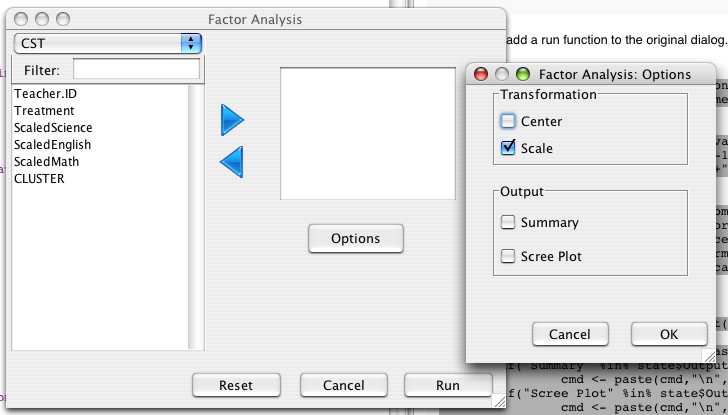The SimpleRSubDialog class is an easy way to add lesser used or advanced options to your GUI.

# Using SimpleRSubDialog

`SimpleRSubDialog` can be used in much the same way as `SimpleRDialog`. let us continue with the 'Factor Analysis' example. In the listeners page we saw how to add an option button to the dialog, which gave us the following code:

```	#make dialog
dialog <- new(SimpleRDialog)
dialog\$setSize(500L,400L)
dialog\$setTitle("Factor Analysis")

variableSelector <- new(VariableSelectorWidget)
variableSelector\$setTitle("data")

#add a list for the variables
variableList<- new(VariableListWidget,variableSelector)
variableList\$setTitle("variables")

JButton <- J("javax.swing.JButton")
button <- new(JButton,"Options")

```

Now lets make a new SimpleRSubDialog and set `dialog` as it's owner (or parent). All sub-dialogs should be linked to another dialog, which can be an RDialog, SimpleRDialog, or another SimpleRSubDialog. When a widget is added to a SimpleRSubDialog, its owner will keep track of it, so that the widget states are remembered and handled correctly.

```	#make Options Dialog
subDialog <- new(SimpleRSubDialog,dialog,"Factor Analysis: Options")
setSize(subDialog,250,300)
```

Now we can add some widgets to the dialog:

```	#options for transforming the variables
transBoxes <- new(CheckBoxesWidget,"Transformation",c("Center","Scale"))
transBoxes\$setDefaultModel(c("Scale"))

#output options
outBoxes <- new(CheckBoxesWidget,"Output",c("Summary","Scree Plot"))
```

Then we add an action listener to the options button to open the sub-dialog when it is pressed:

```	#Listen for the button to be pressed
ActionListener <- J("org.rosuda.deducer.widgets.event.RActionListener")
actionFunction <- function(cmd,ActionEvent){
subDialog\$setLocationRelativeTo(button)
subDialog\$run()
}
listener <- new(ActionListener)
listener\$setFunction(toJava(actionFunction))
```

finally we can add a run function to the original dialog.

```.factorAnalysisRunFunction <- function(state){
#print(state) #a print statement is useful for debugging

#make formula
form <-paste( " ~ " , state\$variables)
for( var in state\$variables[-1])
form <- paste(form,"+",var)

#make prcomp call
cmd <- paste("pr.model <-prcomp(", form, ",", state\$data)
if("Center" %in%state\$Transformation)
cmd <- paste(cmd,", center=TRUE")
if("Scale" %in%state\$Transformation)
cmd <- paste(cmd,",scale=TRUE")
cmd <- paste(cmd,")")

#always print model
cmd <- paste (cmd,"\n","print(pr.model)")

#output summary and plot if asked for
if("Summary" %in% state\$Output)
cmd <- paste(cmd,"\n","summary(pr.model)")
if("Scree Plot" %in% state\$Output)
cmd <- paste(cmd,"\n","screeplot(pr.model)")

#execute command as if typed into console
execute(cmd)
}
dialog\$setRunFunction(toJava(.factorAnalysisRunFunction))
```

Notice that the state of the 'Transformation' and 'Output' widgets are passed to the 'state' parameter. The final result looks as follows: